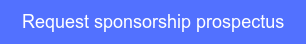<img height="1" width="1" style="display:none" alt="" src="https://www.facebook.com/tr?id=367542720414923&amp;ev=PageView&amp;noscript=1">### 2pm - 3.30pm NZDT## Partners

+6142 4467 120
true true true true true true true true true true true true true true true true true true true true true true true true true true true true true true true true true true true true true true true true true true true true true true true true true true true true true true true true true true true true true true true true true true true true true true true true true true true true true true true true true true true true true true true true true true true true true true true true true true true true true true true true true true true true true true true true true true true true true true true true true true true true true true true true true true true true true true true true true true true true true true true true true true true true true true true true true true true true true true true true true true true true true true true true true true true true true true true true true true